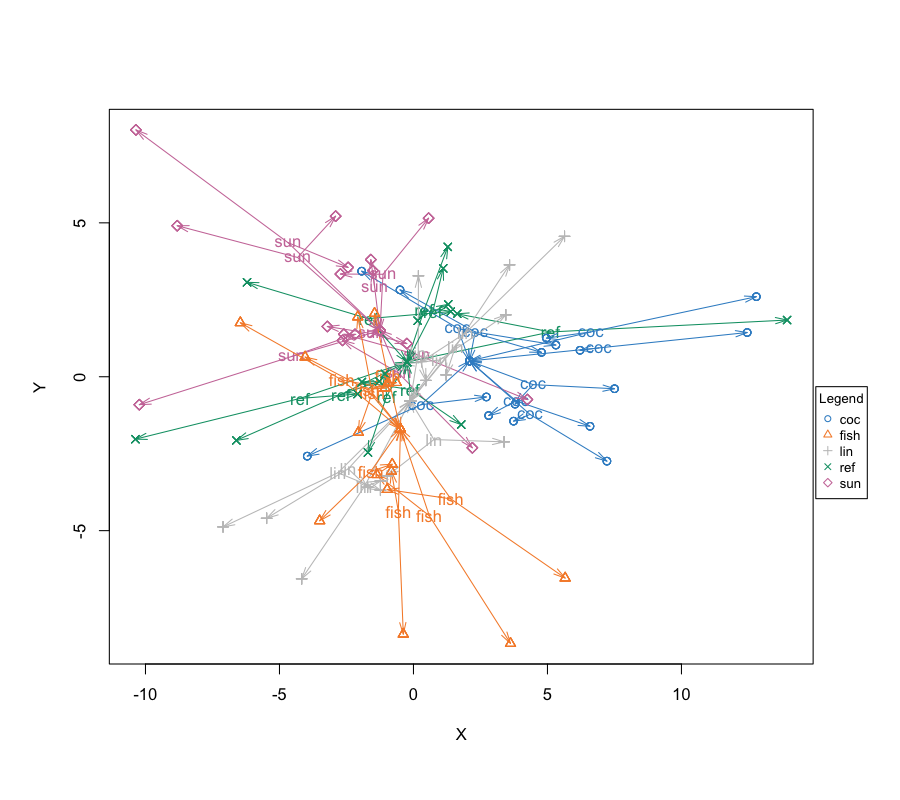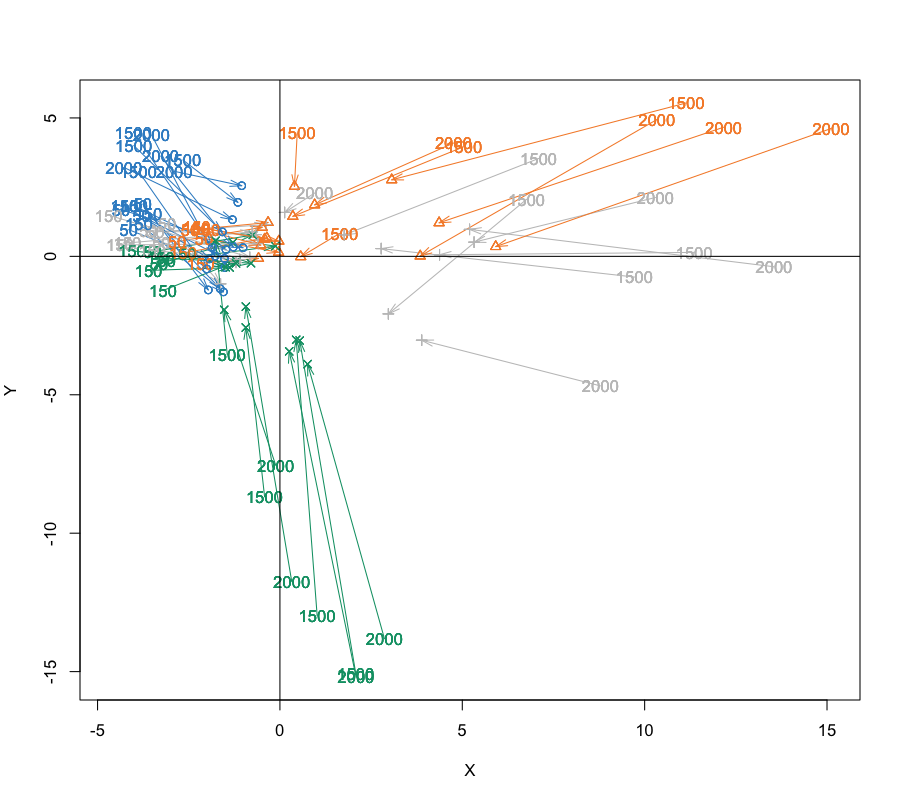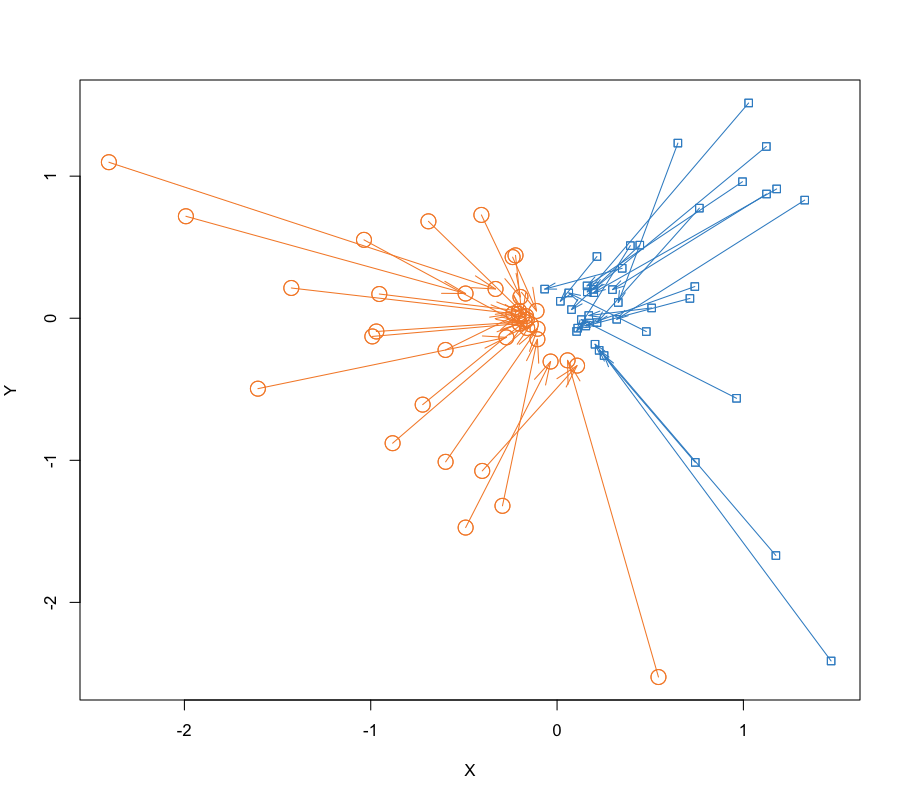# Arrow plot

Each arrow corresponds one sample. The start of the arrow indicates the location of the sample in X in one plot, and the tip the location of the sample in Y in the other plot. Short arrows indicate if both data sets strongly agree and long arrows a disagreement between the two data sets. the latent variables (or ‘variates’) are given as an input from a (s)pls object.

# Usage in mixOmics

library(mixOmics)

#SGCCA

diet = unmap(nutrimouse$diet) blocks = list(gene = nutrimouse$gene,
lipid = nutrimouse$lipid, diet = diet) design = matrix(c(0,1,1,1,0,1,1,1,0), ncol = 3, nrow = 3, byrow = TRUE) nutri.sgcca <- wrapper.sgcca(blocks,design=design, ncomp = 3) #test blocks with ind.names vector and group plotArrow(nutri.sgcca, ind.names= nutrimouse$diet,
group=nutrimouse$diet, legend=TRUE)#PLS/SPLS data(liver.toxicity) X <- liver.toxicity$gene
Y <- liver.toxicity$clinic toxicity.spls <- spls(X, Y, ncomp = 3, keepX = c(50, 50, 50), keepY = c(10, 10, 10)) ###position start , group and abline plotArrow(toxicity.spls, ind.names= liver.toxicity$treatment[, 3],
position.names='start',
group= liver.toxicity$treatment[, 4], abline = TRUE)#RCC data(nutrimouse) X <- nutrimouse$lipid
Y <- nutrimouse$gene nutri.res <- rcc(X, Y, ncomp = 3, lambda1 = 0.064, lambda2 = 10) plotArrow(nutri.res, col = color.mixo(as.numeric(nutrimouse$genotype)),
pch=c(0,1),
cex=c(1,2))